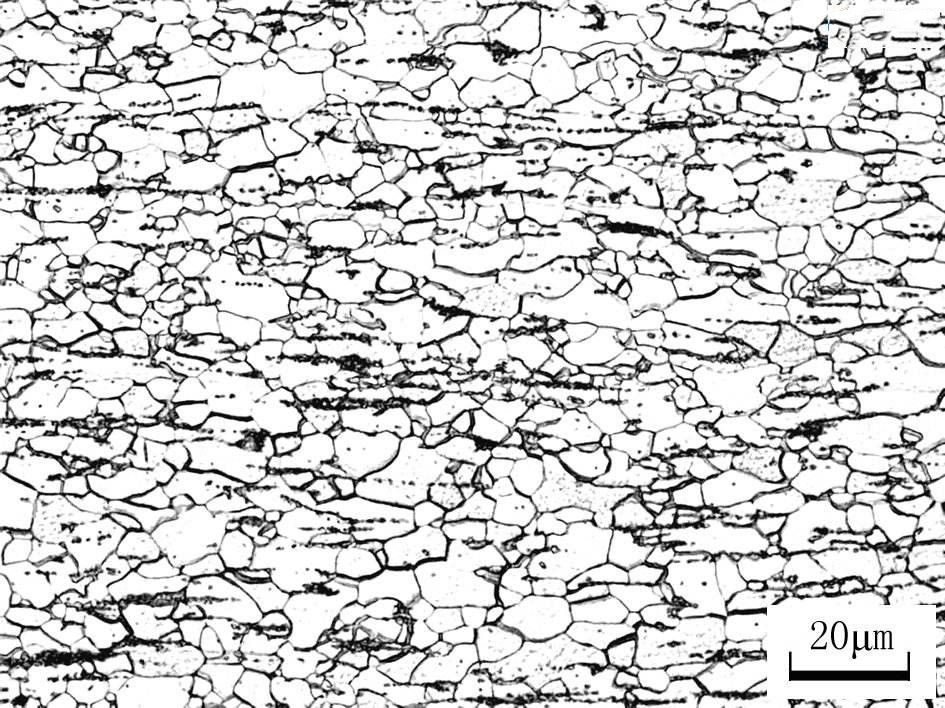# Measuring Method of Grain Size

05/05/2022
Grain size is the scale of grain size. The grain size of metal has a great influence on many properties of metal. The effect of grain size is essentially the effect of grain boundary area. The smaller the grain size, the larger the grain boundary area and the greater the influence on the properties. For the mechanical properties of metal at room temperature, the finer the grain, the higher the strength and hardness, and the better the plasticity and toughness.measuring method

1. Determine the magnification of the photo
First measure the size, length or width of the micrograph, and then measure the actual length or width of the sample
Magnification = picture distance / actual distance

2. Find the number of grain size grades
After calculating the magnification, the number of grain size levels can be determined. First, calculate the number of grains in the sample.
Number of grains = number of complete grains + 0.5 times of partial grains. The grain boundaries of intact grains are observable.
Secondly, calculate the actual area. The actual area = picture length / magnification x width / magnification
According to the calculation formula in ASTM standard: n = 2 (n-1), where n refers to the number of grains per square inch at 100 times magnification and N refers to the number of grain grades. After unit conversion, the value of N can be obtained. Finally, the number of grain grades n can be calculated.

3. Calculate the average grain diameter
Average grain diameter = actual length of the specimen / number of grains in the intercepted part
Actual length = section length / magnification